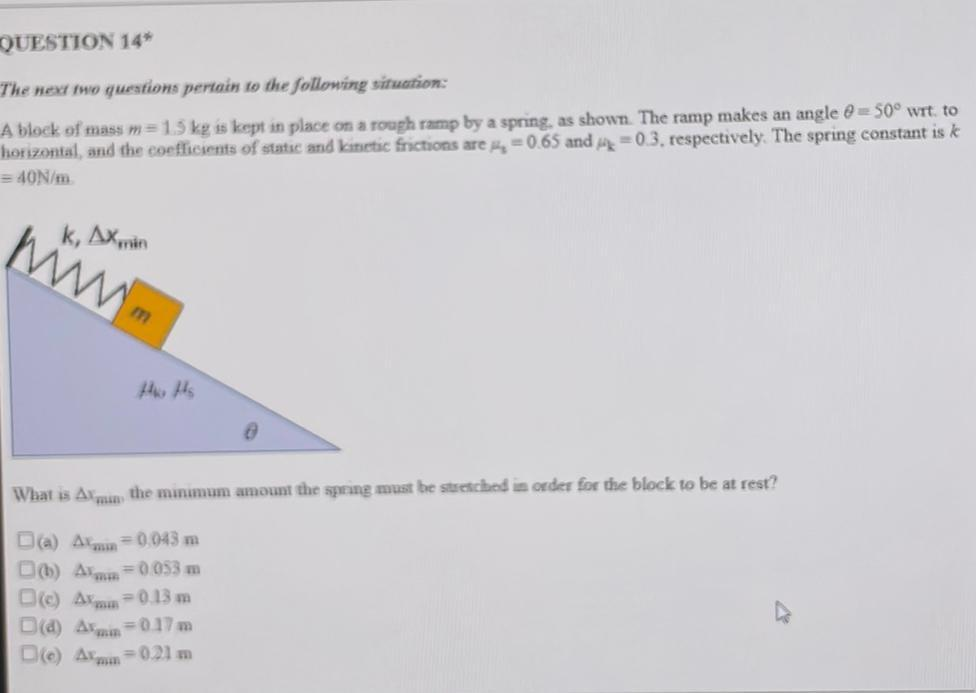Question:

# QUESTION 14* The next to questions pertain to the following situation: A block of mass m= 1.5 kg is kept in place on a rough ramQUESTION 14* The next to questions pertain to the following situation: A block of mass m= 1.5 kg is kept in place on a rough ramp by a spring, as shown. The ramp makes an angle 0 = 50° wrt, to horizontal, and the coefficients of stats and kinetic frictions are =0.65 and 14 = 0.3, respectively. The spring constant is k = 40N/m rinn k, AXmrin @ What is Arman, the minimum amount the spring must be stretched in order for the block to be at rest? D(a) Alan = 0,043 m D() Arm=0053 m = 0.13 m D(a) Armin=0.17 m = 0.21 m De Amo D Am